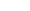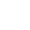# 公設化集合論的奧秘(11) 探索神奇的實數尺寸

2015/02/10 | | 標籤：π/2 (a x +b) =y

r1 = 0.320059874…

r2 = 0.912533121…

r3 = 0.007213568…

r4 = 0.552418792…

r5 = 0.778451420…

r6 = 0.118841234…

r7 = 0.665590012…

x = 0.1211121…

## 關於作者